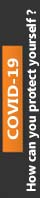Jul 22, 2017 Research papers

# momentum of an object

This paper concentrates on the primary theme of momentum of an object in which you have to explain and evaluate its intricate aspects in detail. In addition to this, this paper has been reviewed and purchased by most of the students hence; it has been rated 4.8 points on the scale of 5 points. Besides, the price of this paper starts from £ 40. For more details and full access to the paper, please refer to the site.

# Discussion: momentum of an object

INSTRUCTIONS:

Please read the discussions below, and tell what you really like about each one. Make sure you understand the physics involved. Please say positive things and keep them separate.

I would not recommend anyone short of superman, to try and stop a car that is moving the same speed as a mosquito with their bare hand. Momentum (p) is the product of the mass (m) and velocity (v) or an object. Without going into detail, it is easy to see that the mass of a mosquito is an awful lot smaller than that of a car. Therefore just based off this formula the momentum of a 100kg car is much bigger than a 2.5 mg mosquito. However, say an individual is convinced that they are superman, and need to be talked out of attempting to stop the car; there are multiple equations and theorems that are useful in doing so. The momentum of  the mosquito is ( 2.5 x 10^-6 kg)  (3m/s) =.0000075 or 7.5x 10^-6 kg*m/s. This is clearly an extremely small momentum, and because of this is would be easy to stop a mosquito while it is in flight. The momentum of a small car is (1000kg) (3 m/s)=3000 kg*m/s. This is clearly a lot bigger of a number which would make it harder to stop it. If you take this and solve for momentum and kinetic energy, you would use the formula k=1/2(m)(v)^2=1/2(m)(p/m)^2. So 1/2(2.5x10-6) (7.5x10-6/2.5x10-6)^2 =1.125x10-27 for the mosquito. The small car in regards to the same formula, is 1/2 (1000)(3000/1000)^2=4500. As we look again at the differences in kinetic energy that it takes to move these two, we`ll see that it takes a very miniscule amount of force to create movement for the mosquito, and a very large amount of force to do the same for the car. This can be thought of in respect to its opposite reaction; it would take a large amount of force to cause the car to stop, and a small to cause the mosquito.

Impulse states that; when a force acts on an object, the objects change in momentum depends on the force and on the time interval which it acts. In regards to our discussion; the force omitted by the person must be enough to slow the car or mosquito from 3m/s to 0m/s. Well use the formula, J=m(Vfx-Vix); we do not have to worry about the y-axis because there is none in this problem. Let`s plus our numbers in J=1000kg(0m/s-3m/s)= -3000J. The force that it would take to stop the car is a force that isn`t possible for the average person. In comparison with the mosquito; again let`s plug in our numbers. J=(2.5x10^-6kg)(0m/s-3m/s)=-7.5x10^-6. To avoid confusion the negative sign states the direction of the force

Momentum of an object is the product of its mass and velocity. Momentum of an object tends to be in the same direction of velocity. The average mass is about 2.5 mg so about 2.5x10-6  kg. The average velocity of a mosquito is around 0.67 m/s. This means that the average momentum for a mosquito is 1.8x10-6 kg(m)/s.  The average mass of a car is around 2000kg. If this car was moving at 0.67 m/s its momentum would be 1340 kg(m)/s. So even though the car is moving at the same velocity of the car, its greater mass means that it has a higher momentum. There would not be any way for a person to stop this car with their hand.

CONTENT:
Discussion: momentum of an objectStudent:Professor:Course title:Date:Discussion: momentum of an objectDiscussion 1: This first discussion is very enlightening as it clearly contrasts the momentum of a car and that of a mosquito. The momentum of a small vehicle is (1000kg) (3 m/s)=3000 kg*m/s, while that of a mosquito is just (2.5 x 10^-6 kg)Â (3m/s) =.0000075 or 7.5x 10^-6 kg*m/s. Given that the momentum of a mosquito is considerably smaller relative to that of a car, it is very easy to stop a mosquito while it is in flight, and it would be harder to ...

0% Plagiarism Guaranteed & Custom Written, Tailored to your instructions

International House, 12 Constance Street, London, United Kingdom,
E16 2DQ

## STILL NOT CONVINCED?

We've produced some samples of what you can expect from our Academic Writing Service - these are created by our writers to show you the kind of high-quality work you'll receive. Take a look for yourself!FLAT 25% OFF ON EVERY ORDER.Use "FLAT25" as your promo code during checkout